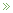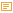• ## 100 Names of Pattern Analysis

Posted by Konstantin 22.10.2008 No Comments

A beloved child has many names, and so does the field of pattern analysis. Some people call it soft computing, others refer to it as machine learning or data-driven statistics. Although in practice all those terms denote the same approach to data analysis, there is always a certain bias hidden behind each name that relates it to its history and origins. I'm not sure whether I understand these biases correctly, but here's how I would define the terms:

Pattern Analysis
This is probably the most general term that refers to a kind of data analysis, where the goal is to find "something interesting" in a given dataset. We typically know what kind of things (patterns) we consider interesting (this might be an association rule, a frequent subsequence, a classifier, etc), and the task is to detect the instances of this kind of patterns. Conceptually somewhat opposite to pattern analysis would be statistical hypothesis testing, where the task is to test a given pattern for interestingness. In this sense pattern analysis is very close to the multiple hypothesis testing statistical problem.
The simplest way to formalize this generic notion I've seen in this exposition by Tilj de Bie. Letdenote the dataset, let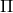be the pattern space (e.g. "the space of all sequences", or "the space of all classifiers", etc), and let for each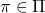there be a pattern function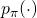, so that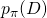measures the "interestingness" of this pattern with respect to the data. Then the general task of pattern analysis is just the following maximization problem: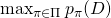Machine Learning
Machine learning can be regarded as a specific type of pattern analysis, where the central interest lies in finding classifiers or regression functions. The dataset in this case consists of a number of "instances"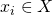, and the task is to find a function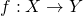that maps these instances to corresponding "outputs"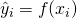in various ways.
In the case of supervised machine learning, a "true" output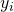is provided with each instance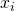, and the resulting function should aim to match this output for given instances (i.e.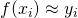) as well as generalize to the unseen ones. Statisticians call this task regression analysis. A classical example is the problem of detecting incoming spam mail by learning the classification rule from previously labeled messages.
The task of unsupervised machine learning is to find a classifier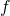without any prespecified labeling of data. Typically one searches for a function that maps similar inputs to similar outputs. If the set of outputs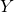is small and discrete, the task is referred to as clustering and somewhat resembles the problem of quantization. If the outputs are continuous, the task can often be related to either factor analysis or noise reduction.
Finally, the problem of semi-supervised machine learning is to find a classifier for a dataset where some instances have labels and some don't. This can sometimes be regarded as task of missing value imputation.
The typical approach to all types of machine learning task usually employs the definition of a loss measure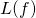, that estimates the goodness of fit for a given classifier, and then searching for a classifier that optimizes this measure, i.e.:This defines machine learning as a certain kind of pattern analysis.

Soft Computing
If the previous terms denote purely mathematical approaches, for which the exact computational method of obtaining a solution is somewhat irrelevant, then this term, on the contrary, denotes a set of certain metaheuristical computational techniques for obtaining these solutions. In the narrowest sense, by soft computing one might collectively denote the areas of evolutionary algorithms, fuzzy logic and neural networks. This gives soft computing a somewhat ad-hockish flavour: "If you don't know how to solve it, simply put it into a neural network, neural networks just work".

Data Mining
This might be just my opinion, but I define data mining as any kind of pattern analysis, that is applied in an offline setting. Moreover, I'd say that, ideally, true data mining happens when you attempt to search for patterns in data that was not initially meant for that specific analysis, thus contradicting the classical statistical ideology "specify your hypothesis first, collect the data later".

Knowledge Discovery from Databases
As far as I understand it, KDD is just a YABA and marketingspeak for "data mining", somewhat in the same manner as OLAP and Business Intelligence are marketingspeak for "descriptive statistics".

Data-Driven Statistics
In the narrowest sense, data-driven statistics denotes all kinds of nonparametric statistical approaches. These are those methods, where one manages to perform data analysis without specifying any parameterized distributions for the inputs or the like. Typical examples would be the various resampling and randomization techniques. In the broad sense, again, this might very much be the same as pattern analysis in general.

Any other popular terms for the same thing I've forgotten here?

### Calendar

October 2008
M T W T F S S
« Sep   Nov »
12345
6789101112
13141516171819
20212223242526
2728293031